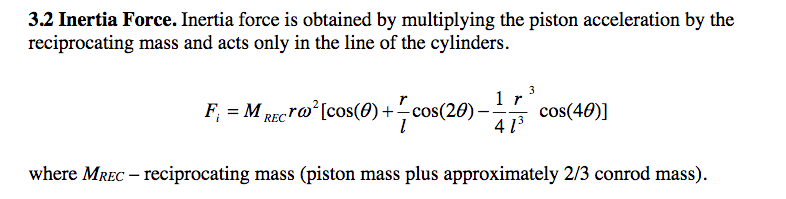# Please identify this physics symbol

• I
jaguar57
I need to know what the w is in this formula.
Also I am not 100% sure which angle it is using.
This is for figuring the inertia of the piston assembly in a 2 stroke engine.
thanks#### Attachments

Homework Helper
Hello jag,I'm pretty sure the ##\omega## is the angular speed of the crankshaft and ##\theta## is its angle. If you could provide some more context, maybe we can settle this with more certainty.

jaguar57
I'm making a spreadsheet and part of it needs to know the piston inertia every 15 degrees.
By "angular speed" do you mean the rotational speed of the crank where the connecting rod connects to it?
And the angle is from the vertical axis?

Mentor
2021 Award
I need to know what the w is in this formula.
Also I am not 100% sure which angle it is using.
This is for figuring the inertia of the piston assembly in a 2 stroke engine.
thanksView attachment 226566
The w is the lower case Greek omega: ##\omega##. It is usually used for angular frequency which in SI units is measured in radians/second

•russ_watters
jaguar57
Since cos(0)=1 and cos(90)=0 then I guess I can assume that the angle of the crank is from the horizontal axis since near 90 and 270 degrees (assuming o degrees is with the piston at the top of its movement) the piston has the highest speed?
Do I need to have all weights in kg and all lengths in meters? (I prefer the metric system)

jaguar57
this is how I made the formula but it's not working for me:
PISTON INERTIA = (piston assembly kg + .66 x con rod kg) x (piston stroke meters/2) x (radians(crankshaft angle change)/second)^2 x COS(crank angle) + ((piston stroke meters/2)/(connecting rod meters)) x COS(2 x crank angle) - ((.5 x piston stroke meters)^3 / (4 x (connecting rod meters)^3) x COS(4 x crank angle))

jaguar57
I got it to work after seeing I forgot to put a pair of parentheses. Here it is corrected:
PISTON INERTIA = (piston assembly kg + .66 x con rod kg) x (piston stroke meters/2) x ((radians(crankshaft angle change per second)^2 x COS(crank angle) + ((piston stroke meters/2)/(connecting rod meters)) x COS(2 x crank angle) - ((.5 x piston stroke meters)^3 / (4 x (connecting rod meters)^3) x COS(4 x crank angle)))

And it did work using the conventional angling of the crank with 0 degrees being with the piston at the top of its stroke.
TDC inertia for my engine is 287 (after converting N/m2 to lbf) and -186 at BDC. But that doesn't agree with the Wallace Racing piston inertia calculator at http://www.wallaceracing.com/Calculate-Inertia-Force-of-Piston.php